UPSC  >  Interests: Solved Examples- 2

# Interests: Solved Examples- 2 - CSAT Preparation - UPSC

Let's have a look at basic formulas before going into Solved questions and answers on Interests.

All interest formulas use the following variables: P = starting principle; r = annual interest rate; t = number of years.

1) Simple Interest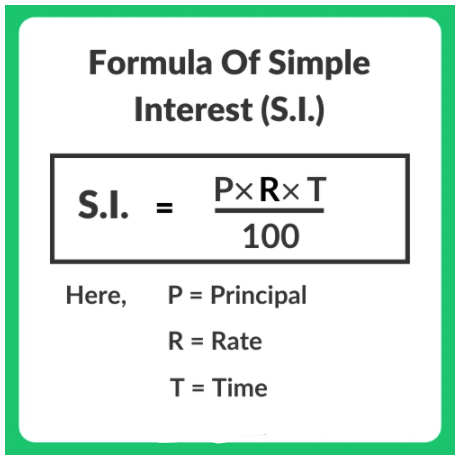2) Compound Interest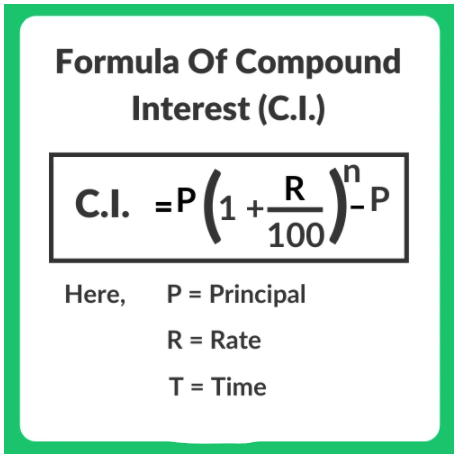## Solved Questions

Question 1: A father left a will of Rs.35 lakhs between his two daughters aged 8.5 and 16 such that they may get equal amounts when each of them reaches the age of 21 years. The original amount of Rs.35 lakhs has been instructed to be invested at 10% p.a. simple interest. How much did the elder daughter get at the time of the will?
(1) Rs. 17.5 lakhs
(2) Rs. 21 lakhs
(3) Rs. 15 lakhs
(4) Rs. 20 lakhs

Explanation: Let Rs.x be the amount that the elder daughter got at the time of the will. Therefore, the younger daughter got (3,500,000 - x).
The elder daughter’s money earns interest for (21 - 16) = 5 years @ 10% p.a simple interest

The younger daughter’s money earns interest for (21 - 8.5) = 12.5 years @ 10% p.a simple interest.
As the sum of money that each of the daughters get when they are 21 is the same,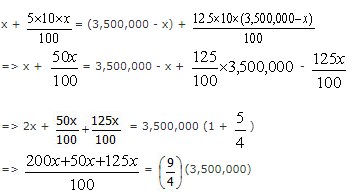=> x = 2,100,000 = 21 lakhs.

Question 2: What will Rs.1500 amount to in three years if it is invested in 20% p.a. compound interest, interest being compounded annually?
(1) 2400
(2) 2592
(3) 2678
(4) 2540

Explanation: The usual way to find the compound interest is given by the formula A =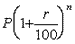.

In this formula, A is the amount at the end of the period of investment
P is the principal that is invested
r is the rate of interest in % p.a
And n is the number of years for which the principal has been invested.
In this case, it would turn out to be A =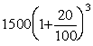So great. How do you find the value of the above term? It is time consuming.
Let us look at another alternative.
What happens in compound interest?
Interest is paid on interest.
In the first year, interest is paid only on the principal. That is very similar to simple interest.
However, from the second year onwards things change. In the second year, you pay
interest on the principal and also interest on interest.
Therefore, the Amount at the end of 2nd year in compound interest can be computed as follows
1 * Principal + 2* Simple interest on principal + 1 * interest on interest.
Similarly, if you were to find the Amount at the end of 3 years in compound interest use the following method
1*Principal + 3 * Simple interest on principal + 3 * interest on interest + 1 * interest on interest on interest
Let us see how it works in our example.
The principal is Rs.1500. The rate of interest is 20%. Therefore, the simple interest on principal is 20% of 1500 = Rs.300
The interest on interest = 20% interest on the interest of Rs.300 = 20% of Rs.300 = Rs.60.
Interest on interest on interest = 20% of Rs.60 = Rs.12.
Amount at the end of 3 years = 1*Principal + 3 * Simple interest on principal + 3 * interest
on interest + 1 * interest on interest on interest
= 1500 + 3*300 + 3*60 + 1*12 = 1500 +900 + 1800 +12 = 2592.
You will get the same answer if you had used the formula. However, the calculation in this case was far easier than using the formula.
Try out the same method for four and five years and remember the 1-2-1, 1-3-3-1, 1-4-6-4-1
the etc method which you can use comfortably in the exam.

Question 3: If a sum of money grows to 144/121 times when invested for two years in a scheme where interest is compounded annually, how long will the same sum of money take to treble if invested at the same rate of interest in a scheme where interest is computed using simple interest method?
(1) 9 years
(2) 22 years
(3) 18 year
(4) 33 years

Explanation: The sum of money grows to  times in 2 years.
If P is the principal invested, then it has grown to P in two years when invested in compound interest.
In compound interest, if a sum is invested for two years, the amount is found using the following formula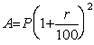= 144/121 P in this case.
=>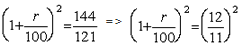=>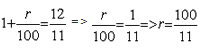If r = 100/11 % then in simple interest the time it will take for a sum of money to treble is found out as follows:
Let P be the principal invested. Therefore, if the principal trebles = 3P, the remaining 2P has come on account of simple interest.
Simple Interest = Pnr/100 , where P is the simple interest, r is the rate of interest and ‘n’ is the number of years the principal was invested.
Therefore, 2P =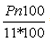=> 2 =  n/11 or n = 22 years.

Question 4: The population of a town was 3600 three years back. It is 4800 right now. What will be the population three years down the line, if the rate of growth of population has been constant over the years and has been compounding annually?
(1) 6000
(2) 6400
(3) 7200
(4) 9600

Explanation: The population grew from 3600 to 4800 in 3 years. That is a growth of 1200 on 3600 during three-year span.
Therefore, the rate of growth for three years has been 1200/3600 = 1/3
The rate of growth during the next three years will also be the same.
Therefore, the population will grow from 4800 by 4800*1/3  = 1600
Hence, the population three years from now will be 4800 + 1600 = 6400.

Question 5: A man invests Rs.5000 for 3 years at 5% p.a. compound interest reckoned yearly. Income tax at the rate of 20% on the interest earned is deducted at the end of each year. Find the amount at the end of the third year.
(1) 5624.32
(2) 5630.50
(3) 5788.125
(4) 5627.20

Explanation:  5% is the rate of interest. 20% of the interest amount is paid as tax. That is 80% of the interest amount stays back. Therefore, if we compute the rate of interest as 80% of 5% = 4% p.a., we will get the same value.
The interest accrued for 3 years in compound interest = 3*simple interest on principal + 3*interest on simple interest + 1*interest on interest on interest. = 3*(200) + 3*(8) + 1*0.32 = 600 + 24 + 0.32 = 624.32
The amount at the end of 3 years = 5000 + 624.32 = 5624.32

Question 6: The difference between the compound interest and the simple interest on a certain sum at 12% p.a. for two years is Rs.90. What will be the value of the amount at the end of 3 years?
(1) 9000
(2) 6250
(3) 8530.80
(4) 8780.80

Explanation:  The difference in the simple interest and compound interest for two years is on account of the interest paid on the first year's interest when interest is reckoned using compound interest, interest is compounded annually.
Hence 12% of simple interest = 90 => simple interest = 90/0.12 =750.
As the simple interest for a year = 750 @ 12% p.a., the principal = 750/0.12 = Rs.6250.
If the principal is 6250, then the amount outstanding at the end of 3 years = 6250 + 3(simple interest on 6250) + 3 (interest on simple interest) + 1 (interest on interest on
interest) = 6250 + 3(750) + 3(90) + 1(10.80) = 8780.80.

Question 7: Vijay invested Rs.50,000 partly at 10% and partly at 15%. His total income after a year was Rs.7000. How much did he invest at the rate of 10%?
(1) Rs.40,000
(2) Rs.10,000
(3) Rs.12,000
(4) Rs.20,000

Explanation: The best way to solve this problem is by using the concept in Mixtures and Alligation.
Vijay earned a total income of Rs.7000 for his investment of 50,000.
Therefore, his average rate of return = 7000/50000 * 100
By rule of allegation, if the value of one of the products is 10 (cheaper) and the other is 15 (dearer) and if the two are mixed such that the average value of the mixture is 14 (mean price), then the two products have been mixed in the following ratio.
The ratio of
Cheaper product: Dearer product
(Dearer product price - mean price) : (Mean price - cheaper product price)
In our example, the cheaper product is the investment at 10%, the dearer product is the
investment at 15% and the mean price is the average return of 14%.
Therefore, the amount invested @10% interest = 1/5 * 50,000 = 10,000.

Question 8: A sum of money invested for a certain number of years at 8% p.a. simple interest grows to Rs.180. The same sum of money invested for the same number of years at 4% p.a. simple interest grows to Rs.120 only. For how many years was the sum invested?
(1) 25 years
(2) 40 years
(3) 33 years and 4 months
(4) Cannot be determined

Explanation:
From the information provided we know that,
Principal + 8% p.a. interest on principal for n years = 180 …….. (1)
Principal + 4% p.a. interest on principal for n years = 120 ……… (2)
Subtracting equation (2) from equation (1), we get
4% p.a. interest on principal for n years = Rs.60.
Now, we can substitute this value in equation (2),
i.e Principal + 60 = 120
= Principal = Rs.60.
We know that SI =  pnr/100, where p is the principal, n the number of years and r the rate percent of interest.
In equation (2), p = Rs.60, r = 4% p.a. and the simple interest = Rs.60.
Therefore, 60 = (60*n*4)/100
=> n = 100/4 = 25 years.

Question 9: How long will it take for a sum of money to grow from Rs.1250 to Rs.10,000, if it is invested at 12.5% p.a simple interest?
(1) 8 years
(2) 64 years
(3) 72 years
(4) 56 years
Explanation:

Simple interest is given by the formula SI = (pnr/100), where p is the principal, n is the number of years for which it is invested, r is the rate of interest per annum
In this case, Rs. 1250 has become Rs.10,000.
Therefore, the interest earned = 10,000 – 1250 = 8750.
8750 = [(1250 x n x 12.5)/100]
=> n = 700 / 12.5 = 56 years.

Question 10: Rs. 5887 is divided between Shyam and Ram, such that Shyam's share at the end of 9 years is equal to Ram's share at the end of 11 years, compounded annually at the rate of 5%. Find the share of Shyam.

(1) 2088
(2) 2000
(3) 3087
(4) None of these
Explanation:

Shyam's share * (1+0.05)9 = Ram's share * (1 + 0.05)11
Shyam's share / Ram's share = (1 + 0.05)11 / (1+ 0.05)9 = (1+ 0.05)2 = 441/400
Therefore Shyam's share = (441/841) * 5887 = 3087.

Question 11: The question for the day is from the topic simple and compound interest. Shawn invested one-half of his savings in a bond that paid simple interest for 2 years and received Rs.550 as interest. He invested the remaining in a bond that paid compound interest, interest being compounded annually, for the same 2 years at the same rate of interest and received Rs.605 as interest. What was the value of his total savings before investing in these two bonds?
(1) Rs.5500
(2) Rs.11000
(3) Rs.22000
(4) Rs.2750

Explanation:
Shawn received an extra amount of (Rs.605 – Rs.550) Rs.55 on his compound interest paying bond as the interest that he received in the first year also earned interest in the second year.
The extra interest earned on the compound interest bond = Rs.55
The interest for the first year =550/2 = Rs.275

Therefore, the rate of interest =55/275 * 100 = 20% p.a.
20% interest means that Shawn received 20% of the amount he invested in the bonds as interest.
If 20% of his investment in one of the bonds = Rs.275, then his total investment in each of the bonds = 275/20 * 100 = 1375.
As he invested equal sums in both the bonds, his total savings before investing = 2*1375 = Rs.2750.

Question 12: Rs.100 doubled in 5 years when compounded annually. How many more years will it take to get another Rs.200 compound interest?
(1) 10 years
(2) 5 years
(3) 7.5 years
(4) 15 years
(5) 8 years

Explanation:
Rs.100 invested in compound interest becomes Rs.200 in 5 years.
The amount will double again in another 5 years.
i.e., the amount will become Rs.400 in another 5 years.
So, to earn another Rs.200 interest, it will take another 5 years.

Question 13: In the beginning of the year 2004, a person invests some amount in a bank. In the beginning of 2007, the accumulated interest is Rs.10,000 and in the beginning of 2010, the accumulated interest becomes Rs.25,000. The interest rate is compounded annually and the annual interest rate is fixed. The principal amount is _____:
(1) Rs.16,000
(2) Rs.18,000
(3) Rs.20,000
(4) Rs.25,000
(5) None of the above
Explanation:

Given Data
Interest from the start of 2004 to the start of 2007 (for 3 years) = Rs.10,000

Interest from the start of 2004 to the start of 2010 (for 6 years) = Rs.25,000
Variables Assigned
Let the principal = x
And annual interest rate = 100r%
The formula to compute the Amount when a sum is invested in compound interest is given as follows: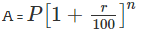Where A is the amount, P is the principal, r is the rate of interest per annum in % and n is the number of years
We have assigned x as the principal and 100r% as the rate of interest.

∴ The amount for 3 years A3 =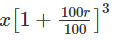= x(1 + r)3 = x + 10000 (Amount = Principal + Interest)
And amount for 6 years A3 = x(1 + r)6 = x + 25000
Further let us take, (1 + r)3 = a
Then, x(1 + r)3 = xa = x + 10000 ..... (1)
And x(1 + r)6 = xa2 = x + 25000 ..... (2)

Solve the two equations to find x
xa = x + 10000 ..... (1)
xa2 = x + 25000 ..... (2)

Bringing x terms to one side in both the equations, we can rewrite the two equations as
x(a – 1) = 10000
x(a2 – 1) = 25000
Equation (2) can therefore be expressed as x(a2 – 1) = x(a - 1) (a + 1) = 25000
We know from equation (1) x(a – 1) = 10000
Substitute x(a – 1) = 10000 in equation (2). ∴ x(a - 1) (a + 1) = 10000 (a + 1) = 25000
Or, (a + 1) = 25000/10000 = 2.5
If a + 1 = 2.5, a – 1 = (a + 1 – 2) = 2.5 – 2 = 0.5
From equation (1) we know x(a – 1) = 10000
We have computed (a – 1) = 0.5
So, x = 10000/0.5 = 20000.

The document Interests: Solved Examples- 2 | CSAT Preparation - UPSC is a part of the UPSC Course CSAT Preparation.
All you need of UPSC at this link: UPSC

## CSAT Preparation

197 videos|151 docs|200 tests

## FAQs on Interests: Solved Examples- 2 - CSAT Preparation - UPSC

 1. What are the eligibility criteria for bank exams?Ans. The eligibility criteria for bank exams vary depending on the specific exam. Generally, candidates must have a minimum educational qualification of a bachelor's degree from a recognized university. Additionally, there may be age limits, nationality requirements, and specific criteria related to work experience or language proficiency. It is advised to refer to the official notification of the exam for detailed eligibility criteria.
 2. How can I apply for bank exams?Ans. To apply for bank exams, candidates need to visit the official website of the respective bank or the organization conducting the exam. They should look for the recruitment or career section and find the specific exam they want to apply for. Then, they need to fill out the online application form, providing all the required information accurately. Along with the application form, candidates may need to upload scanned copies of their photograph, signature, and other necessary documents. It is important to pay the application fee, if applicable, and submit the form within the specified deadline.
 3. What is the selection process for bank exams?Ans. The selection process for bank exams typically involves multiple stages. It generally includes a preliminary examination, a main examination, and an interview (or a group discussion). The preliminary examination is usually a screening test, and candidates who qualify it move on to the main examination. The main examination is more comprehensive and tests the candidate's knowledge in various subjects related to banking and finance. Based on the performance in the main examination, candidates are shortlisted for the interview or group discussion round. The final selection is made considering the candidate's performance in all the stages.
 4. What is the syllabus for bank exams?Ans. The syllabus for bank exams may vary depending on the specific exam and the conducting organization. However, some common subjects/topics that are usually included are: - English Language - Quantitative Aptitude/Numerical Ability - Reasoning Ability - General Awareness/Banking Awareness - Computer Knowledge Candidates are advised to refer to the official notification or exam pattern provided by the conducting organization to get the detailed syllabus and topic-wise breakup for the specific exam they are preparing for.
 5. Are there any negative marks for wrong answers in bank exams?Ans. Yes, there is usually a provision for negative marking in bank exams. For every incorrect answer, a certain amount of marks (usually 1/4th or 1/3rd of the marks allotted to that question) is deducted as a penalty. However, it is important to note that marks are not deducted for unattempted questions. Candidates should carefully evaluate their knowledge and attempt the questions they are confident about to avoid unnecessary negative marking.

## CSAT Preparation

197 videos|151 docs|200 testsExplore Courses for UPSC exam### How to Prepare for UPSC

Read our guide to prepare for UPSC which is created by Toppers & the best Teachers
Signup to see your scores go up within 7 days! Learn & Practice with 1000+ FREE Notes, Videos & Tests.
10M+ students study on EduRev
Track your progress, build streaks, highlight & save important lessons and more!
Related Searches

,

,

,

,

,

,

,

,

,

,

,

,

,

,

,

,

,

,

,

,

,

;